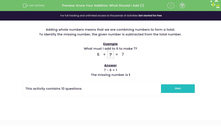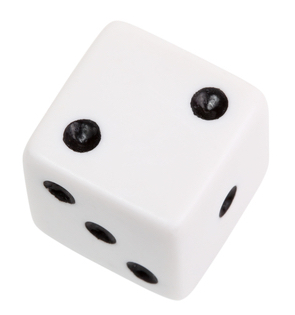### Comprehensive & curriculum aligned

In this worksheet, students will work out what number (from 0-10) they should add to complete a sum.Key stage:  KS 1

Curriculum topic:   Number: Addition and Subtraction

Curriculum subtopic:   Solve Add/Subtract Problems to 20

Difficulty level:#### Worksheet Overview

Adding whole numbers means that we are combining numbers to form a total.

Example:

6 + 1 = 7

From the calculation above, we also know that 1 + 6 = 7

We can also use the calculation to help us solve two subtraction problems:

7 - 1 = 6

7 - 6 = 1

Brilliant stuff, eh?Sometimes, calculations can have gaps in the middle, instead of at the end.

We can work them out by rearranging the order of the calculation.

For example, look at this problem:

6 + ? = 7

This question is asking us what we need to add to 6 to get 7.

If we change the order of the calculation, it becomes much easier to do:

7 - 6 = ?

You know the answer to this one!

7 - 6 = 1

So, the missing number is 1Let's try another one.

What is the mystery number in this calculation?

2 + ? = 4

You can answer this in two ways.

You can count on from 2 to 4. How many numbers did you count on? It was 2, so that's your answer!

Or you can rearrange the order:

4 - 2 = ?

Now you can subtract 2 from 4.  Surprise, surprise, the answer is 2.

4 - 2 = 2

So the mystery number is 2Let's have a go at some questions now.### What is EdPlace?

We're your National Curriculum aligned online education content provider helping each child succeed in English, maths and science from year 1 to GCSE. With an EdPlace account you’ll be able to track and measure progress, helping each child achieve their best. We build confidence and attainment by personalising each child’s learning at a level that suits them.

Get started